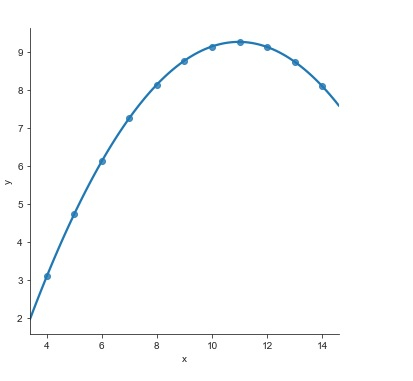# How can a polynomial regression model be fit to understand non-linear trends in data in Python?

When regression models are being built, multicollinearity is checked for. This is because we need to understand the correlation present between all different combinations of continuous variables. If multicollinearity exists between the variables, we have to make sure that it is removed from the data.

The data in real world is usually non-linear. We need to find mechanisms to fit such non-linear data to the model. We will be using Anscombe’s dataset to visualize this data.

The ‘implot’ function is used with non-linear data −

## Example

import pandas as pd
import seaborn as sb
from matplotlib import pyplot as plt
sb.lmplot(x = "x", y = "y", data = my_df.query("dataset == 'II'"),order = 3)
plt.show()

## Output## Explanation

• The required packages are imported.
• The input data is ‘anscombe’ which is loaded from the seaborn library.
• This data is stored in a dataframe.